# PSAT Math : How to find decimal equivalent to a percentage

## Example Questions

### Example Question #1 : Decimals And Percentage

55 and 1/2% of 23 is about what?

2

155

11

49

13

13

Explanation:

55 and 1/2% can be written as a decimal: 0.555. To see what number is about 55.5% of 23, multiply 0.555 by 23. Answer: 12.765 or about 13.

Another route is to say that 55.5% is about half of 23. Half of 23 is 11.5. Since 55.5% is greater than 50%, 13 is the logical choice instead of 11.

### Example Question #2 : Decimals And Percentage

Let x and y be numbers such that x and y are both nonzero, and x > y. If half of x is equal to thirty percent of the positive difference between x and y, then what is the ratio of x to y?

–1

3/2

2/3

–2/3

–3/2

–3/2

Explanation:

We need to find expressions for fifty percent of x and for thirty percent of the positive difference between x and y. Then, we can set these two expressions equal to each other and determine the ratio of x to y.

Fifty percent of x is equal to one-half of x, which is the same as multiplying x by 0.50.

50% of x = 0.5x

Thirty percent of the positive difference between x and y means that we need to multiply the positive difference between x and y by thirty percent. Because x > y, the positive difference between x and y is equal to x – y. We then need to take thirty percent of the quantity x – y. Remember that to convert from a percent to a decimal, we move the decimal two spaces to the left. Therefore, 30% = 0.30. We can now multiply this by (x – y).

30% of x – y = 0.30(x – y)

Now, we set the two expressions equal to one another.

0.5x = 0.30(x – y)

Distribute the right side.

0.5x = 0.3x – 0.3y

The ratio of x to y is represent by x/y. Thus, we want to group the x and y terms on opposite sides of the equations, and then divide both sides by y.

0.5x = 0.3x – 0.3y

Subtract 0.3x from both sides.

0.2x = –0.3y

Divide both sides by 0.2

x = (–0.3/0.2)y

Divide both sides by y to find x/y.

x/y = (–0.3/0.2) = –1.5.

Because the answers are in fractions, we want to rewrite –1.5 as a fraction. We can write –1.5 as –1.5/1 and then mutiply the top and bottom by 2.

(–1.5/1)(2/2) = –3/2

### Example Question #41 : Percentage

If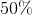ofis equal toof, andofis equal to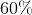of, then what percent ofis?

125

75

100

133

25

75

Explanation:

We are told that 50% of x is equal to 25% of y. We need to represent these two pieces of information as algebraic expressions. We can convert 50% and 25% to decimals by moving the decimals two places to the left. Thus, 50% = 0.50, and 25% = 0.25. To find 50% of x, we multiply x by 0.50. In other words, 50% of x = 0.50x. Likewise, 25% of y = 0.25y. We now set 0.50x and 0.25y equal to one another.

0.50x = 0.25y

Let's divide both sides by 0.25 to get rid of decimals.

2x = y

Next, we are told that 40% of y is equal to 60% of z. We will represent 40% and 60% as 0.40 and 0.60, respectively. Thus, we can write the following equation:

0.40y = 0.60z

Ultimately, we are asked to find x as a percentage of z. This means we want to find an equation with x and z, but not y. If we solve for y in the second equation, and then substitute this value into the first, we can eliminate y.

Let's take the equation 0.40y = 0.60z and divide both sides by 0.40.

y = 1.5z

Now, we can take 1.5z and substitute this for y in the first equation.

2x = 1.5z

In order to find x as a percent of z, we must solve for x in terms of z. This means we must divide both sides of the equation by 2.

x = 0.75z

x is 0.75 times z. We can represent 0.75 as 75%, because in order to convert from a decimal to a percent, we need to move the decimal two spaces to the right. Therefore, if x = 0.75z, then x = 75% of z.

### Example Question #1 : How To Find Decimal Equivalent To A Percentage

To get on the ballot for the student body president at Harding High School, a student must turn in a petition with the signatures of 8% of the students from each of the four classes - freshman, sophomore, junior, and senior. There are 342 freshmen, 312 sophomores, 270 juniors, and 268 seniors enrolled at McKinley.

Tom has a petition with the signatures of 25 students from each of the four classes. Can he get on the ballot with the signatures he has, and if not, why not?

Tom has the signatures he needs to get on the ballot.

Tom cannot get on the ballot yet, because he needs more signatures from both freshmen and sophomores.

Tom cannot get on the ballot yet, because he needs more signatures from freshmen.

Tom cannot get on the ballot yet, because he needs more signatures from members of all four classes.

Tom cannot get on the ballot yet, because he needs more signatures from sophomores.

Tom cannot get on the ballot yet, because he needs more signatures from freshmen.

Explanation:

In order to answer the question, we must find out the percent of each class that has signed Tom's petition, and compare it to 8%.

Freshmen: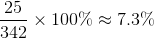have signed.

Sophomores: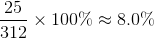have signed.

Juniors: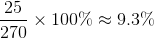have signed.

Seniors: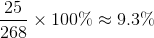have signed.

Tom has the necessary signatures from members of the top three classes, but he cannot get on the ballot yet because he has not gathered enough signatures from freshmen.